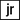# Print a new number by adding one to each of its digit

Project originally posted on reddit.com/r/dailyprogrammer

# Description

A number is input in computer then a new no should get printed by adding one to each of its digit. If you encounter a 9, insert a 10 (don’t carry over, just shift things around).

For example, 998 becomes 10109.

## Solution

``````function addOnes(num) {
const split = num.toString().split('');

return +split.map(each => +each + 1).join('');
}``````

# Bonus

This challenge is trivial to do if you map it to a string to iterate over the input, operate, and then cast it back. Instead, try doing it without casting it as a string at any point, keep it numeric (int, float if you need it) only.

## Solution

``````function addOnesBonus(num) {
const digits = [];

while(num > 0) {
digits[digits.length] = (num % 10) + 1;
num = parseInt(num / 10);
}

return digits.reduce((a, b, ind) => {
return b * Math.pow(10, ind) + a;
}, 0);
}``````